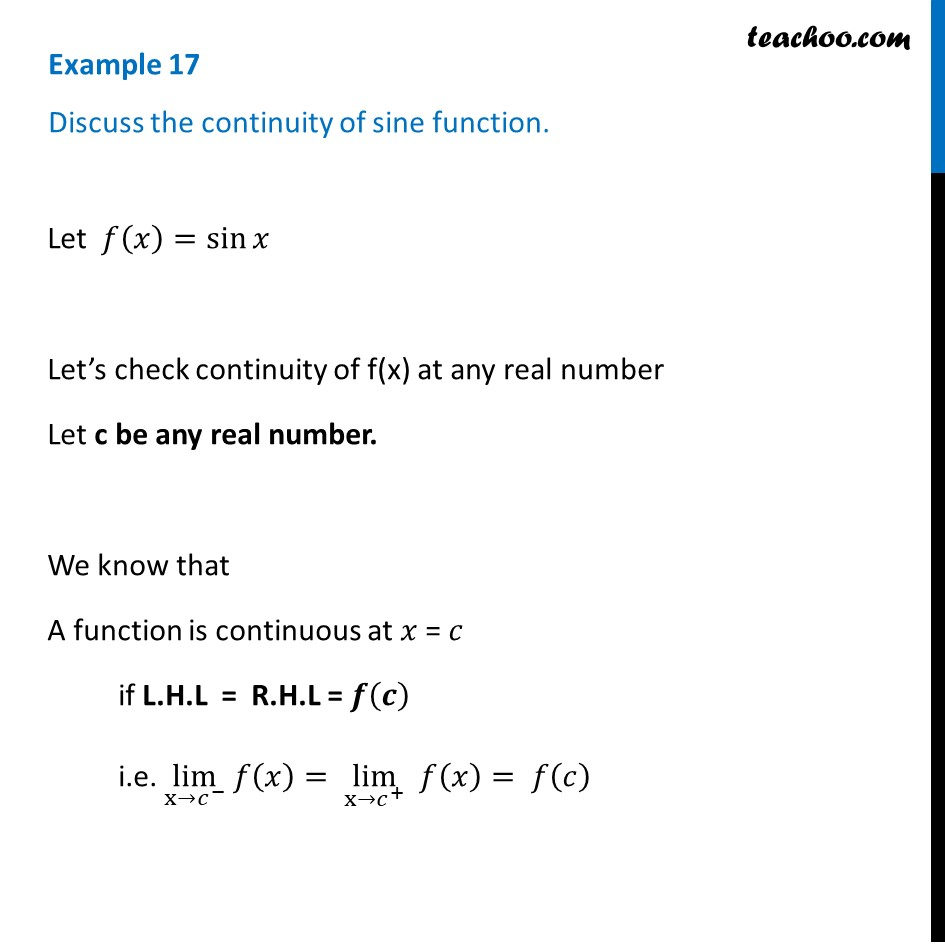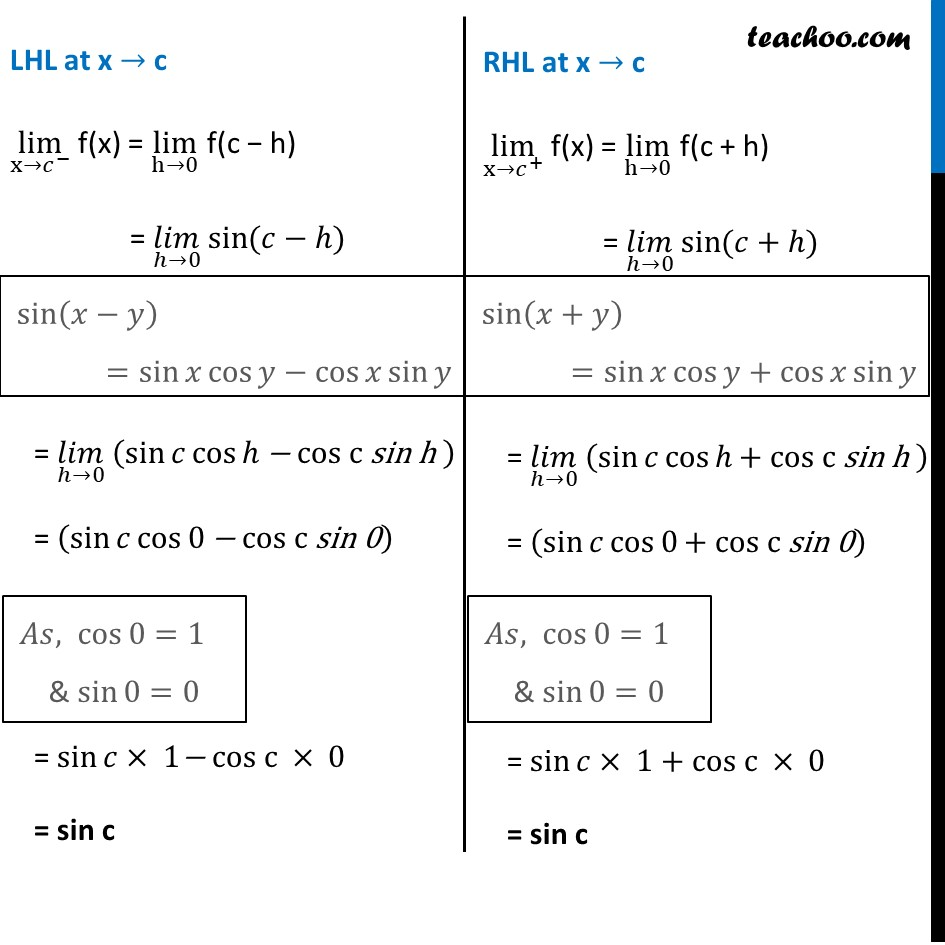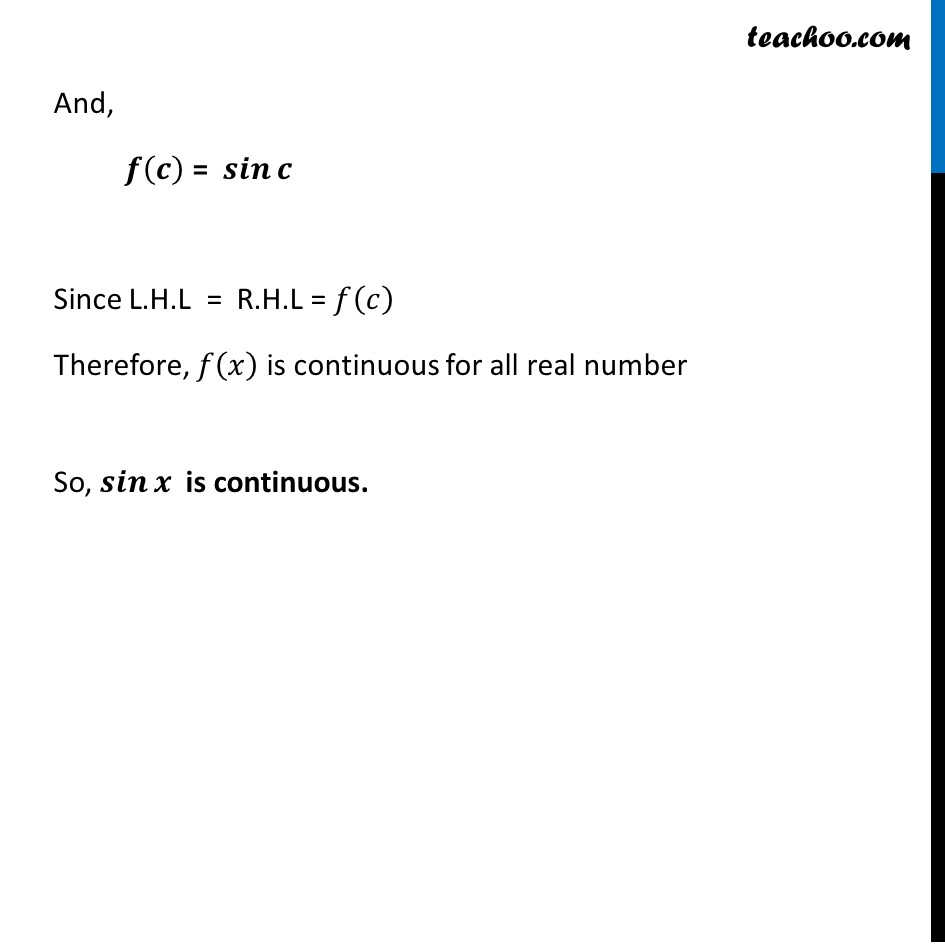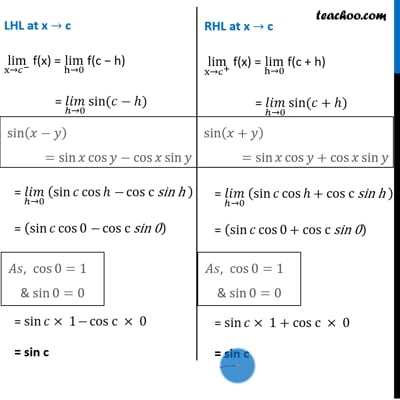Checking continuity at any point

Chapter 5 Class 12 Continuity and Differentiability
Concept wiseThis video is only available for Teachoo black users

Introducing your new favourite teacher - Teachoo Black, at only ₹83 per month

### Transcript

Example 17 Discuss the continuity of sine function.Let 𝑓(𝑥)=sin⁡𝑥 Let’s check continuity of f(x) at any real number Let c be any real number. We know that A function is continuous at 𝑥 = 𝑐 if L.H.L = R.H.L = 𝒇(𝒄) i.e. lim┬(x→𝑐^− ) 𝑓(𝑥)= lim┬(x→𝑐^+ ) " " 𝑓(𝑥)= 𝑓(𝑐) LHL at x → c lim┬(x→𝑐^− ) f(x) = lim┬(h→0) f(c − h) = (𝑙𝑖𝑚)┬(ℎ→0) sin⁡〖(𝑐〗−ℎ) = (𝑙𝑖𝑚)┬(ℎ→0) (sin⁡𝑐 cos⁡ℎ "− cos c sin h " ) = (sin⁡𝑐 cos⁡0 "− cos c sin 0" ) = sin⁡𝑐× 1"− cos c" × 0 = sin c sin⁡(𝑥−𝑦) =sin⁡𝑥 cos⁡𝑦−cos⁡𝑥 sin⁡𝑦 𝐴𝑠, cos⁡0=1 & sin⁡0=0 RHL at x → c lim┬(x→𝑐^+ ) f(x) = lim┬(h→0) f(c + h) = (𝑙𝑖𝑚)┬(ℎ→0) sin⁡〖(𝑐〗+ℎ) = (𝑙𝑖𝑚)┬(ℎ→0) (sin⁡𝑐 cos⁡ℎ "+ cos c sin h " ) = (sin⁡𝑐 cos⁡0 "+ cos c sin 0" ) = sin⁡𝑐× 1" + cos c" × 0 = sin c sin⁡(𝑥+𝑦) =sin⁡𝑥 cos⁡𝑦+cos⁡𝑥 sin⁡𝑦 𝐴𝑠, cos⁡0=1 & sin⁡0=0 And, 𝒇(𝒄) = 𝒔𝒊𝒏⁡𝒄 Since L.H.L = R.H.L = 𝑓(𝑐) Therefore, 𝑓(𝑥) is continuous for all real number So, 𝒔𝒊𝒏⁡𝒙 is continuous.